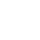NOW ALSO FOR ENGLISH!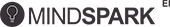# A smart friend that helps maths make sense!

HELLO!

MINDSPARK IS EXCITED TO
HAVE YOU HERE.

I AM A
YOUR CHILD’S A MINDSPARKER,
BUT YOU’RE NEW TO MINDSPARK?

Just fill up the following details
and you’ll be done.

Filling these details will allow you to connect to Mindspark through Parent Connect – a platform where you can access all details on your child’s performance.

OR

Verify OTP

NEW TO MINDSPARK AND
EAGER TO GET STARTED?

This won’t take much time.
All you need to do is give us your
details and you’ll be done.

*Note: Students below 13 need to ask their
parents to create the account.

All fields are compulsory
Subjects:
Subjects:
ARE YOU AND YOUR CHILD
BOTH NEW TO MINDSPARK?

Just a few details and you can get
your child Mindsparking immediately.

Filling these details will allow you to connect to Mindspark through Parent Connect – a platform where you can access all details on your child’s performance.

AND

AN ONLINE TOOL TO ENHANCE CHILDREN'S
UNDERSTANDING OF MATHS

Mindspark is a computer-based, online self-learning tool that helps children improve their Maths skills.
It allows each child to follow a learning path that is based on his or her needs.Urges children to ask questions, develop conceptual understanding and love the subjectHelps educators plan lessons and adopt effective methodologies that aid learning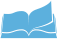Aligned with different curricula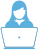80K students use Mindspark regularly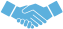More than 200 schools have benefited from Mindspark

A GUIDE THAT WON'T RACE AHEAD OF THE CHILD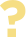Asks questions synchronised with the current learning levelUnderstands a child's learning capacity and calibrates according to itProvides granular content that enables real learning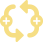Gives in-depth feedback on every response

FUN FEATURES OF MINDSPARK

Exciting, innovative and intuitive features on the Mindspark interface gives students a learning
environment that is personalised, adaptive and interactive.

OUR BACKBONE IS THE MINDFUL IMPLEMENTATION OF
DEEPLY RESEARCHED IDEASNothing can stop you when you immerse yourself in what you do!

The state of flow is the mental state of operation where a person performing an activity is fully immersed in a feeling of energized focus, involvement and enjoyment in the activity.

Practicing on Mindspark helps students enter a state of flow and get fully absorbed in what they are learning, leading them to focus their mental energy on performing better.

A SMART TEACHER’S RELIABLE ASSISTANT

Alignment with the syllabi makes Mindspark easy for teachers to run it as a complementary learning tool.
Detailed individual and grade-wise performance reviews help teachers recognize strengths and weaknesses of their
students and try newer ways of teaching.

## With Mindspark, teachers canGet instant feedback on class performanceGet a detailed view of students’ performanceMake worksheets for practice/testsKeep track of students' activity in real-timeShare and learn from insights from teachers across the country

MINDSPARK IS ALIGNED WITH SCHOOL SYLLABI
Class I Topics

Algebra

• -Patterns

Geometry

• -Shapes

Measurement

• -Length
• -Money
• -Time

Numbers

• -Numbers upto 20
• -Addition upto 20
• -Numbers upto 99
• -Addition upto 99 (without regrouping)
• -Subtraction upto 20
• -More on addition
• -Subtraction upto 99 (without regrouping)
• -More on subtraction
• -Place Value and Addition upto 99 - with regrouping
• -Place Value and Addition upto 999 - with regrouping
• -Multiplication

Statistics and Data Analysis

• -Data handling
Class II Topics

Algebra

• -Patterns

Fractions

• -Fractions

Geometry

• -Shapes

Measurement

• -Length
• -Money
• -Time
• -Mass and capacity

Numbers

• -Numbers upto 20
• -Addition upto 20
• -Numbers upto 99
• -Addition upto 99 - without regrouping
• -Subtraction upto 20
• -Numbers upto 999
• -Addition upto 999 - without regrouping
• -Subtraction upto 99 - without regrouping
• -Subtraction upto 999 - without regrouping
• -Place Value and Addition upto 99 - with regrouping
• -Place Value and Addition upto 999 - with regrouping
• -Place Value and Subtraction upto 99 - with regrouping
• -Place Value and Subtraction upto 999 - with regrouping
• -Multiplication
• -Word problems

Statistics and Data Analysis

• -Data handling
Class III Topics

Algebra

• -Patterns

Geometry

• -Shapes
• -Straight and Curved lines

Measurement

• -Length
• -Money
• -Time
• -Mass and capacity
• -Word problems on Measurement

Numbers

• -Numbers upto 99
• -Numbers upto 999
• -Addition upto 999 - without regrouping
• -Subtraction upto 20
• -Subtraction upto 999 - without regrouping
• -Rounding numbers
• -Place Value and Addition upto 999 - with regrouping
• -Place Value and Subtraction upto 99 - with regrouping
• -Place Value and Subtraction upto 999 - with regrouping
• -Multiplication
• -Laws of Multiplication and more
• -Even and odd numbers
• -Division
• -Word problems

Statistics and Data Analysis

• -Charts and Graphs
Class IV Topics

Algebra

• -Pre-algebra - Developing algebraic reasoning

Fractions

• -Fractions - basic concepts, equivalence and comparison

Geometry

• -Angles
• -Point, lines and planes
• -Symmetry

Measurement

• -Length
• -Money
• -Time
• -Mass and capacity
• -Problems on measurement and estimation

Mensuration

• -Area and perimeter

Numbers

• -Word problems on whole number operations
• -Addition upto 999 (with regrouping)
• -Subtraction upto 999 (with regrouping)
• -Multiplication of numbers
• -Division of numbers
• -Numbers upto 999
• -Addition and subtraction of numbers upto 999
• -Estimation and rounding
• -Even and odd numbers
• -Large numbers
• -Operations on large numbers
• -Properties of whole numbers

Problem solving

• -Problem solving

Statistics and Data Analysis

• -Data representation
Class V Topics

Algebra

• -Pre-algebra - Developing algebraic reasoning

Fractions

• -Fractions - basic concepts, equivalence and comparison
• -Operations on fractions

Decimals

• -Decimals- Fundamentals

Geometry

• -Shapes and space
• -Angles
• -Symmetry

Measurement

• -Length
• -Money
• -Time
• -Mass and capacity
• -Problems on measurement and estimation

Number Theory

• -Factors and multiples
• -Tests of divisibility

Mensuration

• -Volume
• -Area and perimeter
• -Volume and surface areas

Numbers

• -Word problems on whole number operations
• -Addition upto 999 (with regrouping)
• -Order of operations for whole numbers
• -Multiplication of numbers
• -Division of numbers
• -Estimation and rounding
• -Large numbers
• -Operations on large numbers
• -Properties of whole numbers

Problem solving

• -Problem solving

Real numbers

• -Integers

Statistics and Data Analysis

• -Data representation
Class VI Topics

Algebra

• -Algebraic expressions
• -Linear equations in one variable
• -Patterns, relations and functions
• -Sets

Fractions

• -Fractions - basic concepts, equivalence and comparison
• -Operations on fractions

Decimals

• -Decimals- Fundamentals
• -Decimals- Operations

Geometry

• -Triangles and triangle properties
• -Solid shapes
• -Polygons
• -Shapes and space
• -Angles
• -Point, lines and planes
• -Parallel lines
• -Circles and circle properties
• -Symmetry
• -Similarity

Mensuration

• -Area and perimeter
• -Area of plane figures

Number Theory

• -Factors and multiples
• -Tests of divisibility

Numbers

• -Word problems on whole number operations
• -Order of operations for whole numbers
• -Estimation and rounding
• -Even and odd numbers
• -Large numbers
• -Operations on large numbers
• -Properties of whole numbers

Percentages and commercial maths

• -Percentages
• -Simple Interest

Ratio-Proportion

• -Unitary method
• -Ratio and Proportion

Real numbers

• -Integers

Statistics and Data Analysis

• -Data handling
• -Averages - Mean, Mode and Median
Class VII Topics

Algebra

• -Formulae
• -Algebraic expressions
• -Linear equations in one variable
• -Patterns, relations and functions
• -Sets

Fractions

• -Operations on fractions

Decimals

• -Decimals- Fundamentals
• -Decimals- Operations

Mensuration

• -Area and perimeter
• -Area of plane figures
• -Area and circumference of circles

Number Theory

• -Tests of divisibility

Percentages and commercial maths

• -Percentages
• -Profit and Loss
• -Simple Interest

Geometry

• -Triangles and triangle properties
• -Solid shapes
• -Congruence of triangles
• -Shapes and space
• -Point, lines and planes
• -Parallel lines
• -Symmetry
• -Pythagoras theorem
• -Similarity

Ratio-Proportion

• -Unitary method
• -Ratio and Proportion

Real numbers

• -Integers
• -Real numbers
• -Exponents and roots

Statistics and Data Analysis

• -Averages - Mean, Mode and Median
• -Probability
Class VIII Topics

Algebra

• -Formulae
• -Linear Inequalities
• -Algebraic expressions
• -Linear equations in one variable
• -Algebraic identities and factorisation
• -Linear equations in two variables
• -Patterns, relations and functions
• -Sets

Mensuration

• -Surface Area
• -Volume
• -Volume and surface areas
• -Area of plane figures

Ratio-Proportion

• -Unitary method
• -Ratio and Proportion

Real numbers

• -Squares, square roots, cubes and cube roots
• -Real numbers
• -Exponents and roots

Fractions

• -Operations on fractions

Percentages and commercial maths

• -Percentages
• -Banking and taxation
• -Profit and Loss
• -Simple Interest
• -Compound interest

Geometry

• -Triangles and triangle properties
• -Solid shapes
• -Polygons
• -Shapes and space
• -Parallel lines
• -Circles and circle properties
• -Symmetry
• -Coordinate geometry

Statistics and Data Analysis

• -Data handling
• -Averages - Mean, Mode and Median
• -Statistics
• -Probability
Class IX Topics

Algebra

• -Formulae
• -Algebraic identities and factorisation
• -Polynomials - zeroes, Remainder theorem and Factor theorem
• -Linear equations in two variables

Mensuration

• -Surface Area
• -Volume
• -Volume and surface areas
• -Area of plane figures

Real numbers

• -Logarithms
• -Real numbers
• -Exponents and roots

Geometry

• -Transformations
• -Triangles and triangle properties
• -Congruence of triangles
• -Vectors
• -Polygons
• -Parallel lines
• -Circles and circle properties
• -Area theorems
• -Coordinate geometry
• -Similarity

Statistics and Data Analysis

• -Data representation
• -Statistics
• -Probability
Class X Topics

Algebra

• -Linear Inequalities
• -Matrices
• -Polynomials - zeroes, Remainder theorem and Factor theorem
• -Linear equations in two variables
• -Arithmetic progressions

Geometry

• -Transformations
• -Triangles and triangle properties
• -Locus
• -Reflection
• -Vectors
• -Circles and circle properties
• -Symmetry
• -Pythagoras theorem
• -Coordinate geometry
• -Similarity

Mensuration

• -Volume
• -Volume and surface areas
• -Area and circumference of circles

Percentages and commercial maths

• -Shares and Dividends
• -Banking and taxation

Ratio-Proportion

• -Ratio and Proportion

Real numbers

• -Real numbers
• -Exponents and roots

Statistics and Data Analysis

• -Statistics
• -Probability

Trigonometry

• -Trigonometric ratios
• -Heights and Distances
GET YOUR CHILD MINDSPARKING

A world class Maths experience just at 3 cent a day

RENEW SUBSCRIPTION

SELECT

PURCHASE NEW SUBSCRIPTION

SELECT

One step and you're good to go!

## Parent Information

Note: After clicking on the “MAKE PAYMENT” button, you will be directed to a secure gateway for payment. After completing the payment process, you will be redirected back to Mindspark.in By purchasing Mindspark, you agree with the Terms and Conditions and Privacy Policy.

Don't want your child to miss out on fun & interesting learning methods? Hit renew!

OR

Registered Parent Email ID
OR

Registered Parent Phone Number

Enter OTP

Note: Users outside India are requested to use their email IDs for validation.

## Parent Information

Help Manual

Select one of the following questions to learn more

• What is Mindspark?
• Who created Mindspark?
• How is Mindspark unique?
• How is Mindspark different from other e-learning programmes?
• How will Mindspark help my child?
• Is Mindspark available for other subjects?
• For which classes is Mindspark appropriate?
• Is Mindspark in line with the syllabus of the boards?
• How can I Subscribe/Register for Mindspark?
• Is it possible for my child to learn by only answering questions?
• Would using Mindspark help my child get more marks?
• How do I know my child's performance in Mindspark?
• In Mindspark, if my child is presented with maths problems in concepts that have not been taught in school, should my child still continue to do them?
• If my child gets a number of questions he/she says are simple, what should I do?
• My child says many questions are repeated. What should I do?
• What happens after my child has finished all the topics assigned to her?
• Does my child need to do Mindspark everyday?
• Why do some of the questions take more time to appear?
• Will there be page loading issue at home?
• What to do when not able to login or the error message 'Session Expired' appears?
• Is there an ideal browser for using Mindspark?
• What should be done if I face any problem while using Mindspark?
• Why is a correct answer marked wrong by Mindspark?
• Why does the topic progress not move ahead?
• Does Mindspark work on iPad?
• What logic does Mindspark use to award Sparkies?
• What is the Sparkie logic in CQs (Challenge questions) in Mindspark Maths?
• How is the 'Sparkie Champ of the Week' selected?
EDUCATIONAL INITIATIVES

Educational Initiatives (EI) is an edtech company that leverages the twin levers of cutting-edge research and technology-based solutions approach to revolutionise how children engaged in the K-12 education space learn. Our vision is to create a world in which children everywhere learn with understanding. Our goal is to reach a multitude of children across different strata of society, irrespective of the kind of education they are receiving today or the curriculum they are following.

We achieve this goal by building products that focus on diagnostic assessment and personalised learning. Our platforms cull relevant and customised intelligence from over five billion data points to deliver content in the form of questions, activities, games and videos to assess students‘ learning levels and provide explanations, feedback and learning inputs for all stakeholders. This data treasure has been built over two decades and is one-of-its-kind in the education industry.

We believe in a scientific approach to conceptualising, designing and building our products.This stems from a dream to discover SCIENCE OF LEARNING that can systematically be made available to teachers. EI's mission is to build this ‘Science of Learning’ which provides a repository of data and techniques that can be used by teachers to help children learn better - a big improvement over today's situation where teachers struggle alone to help students learn. EI's work on assessment and learning has already helped create banks of misconceptions, common student errors and the like and these are being shared as usable insights.